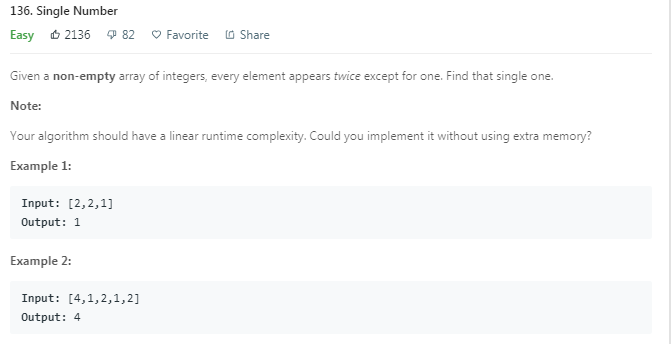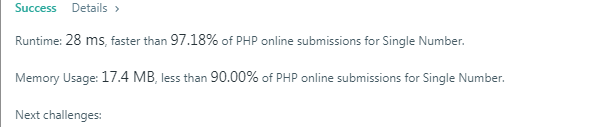# Leetcode之PHP版题目解析(136. Single Number)

Leetcode · curry · 于 2个月前 发布 · 102 次阅读### 题目思路

``````/**
* @param Integer[] \$nums
* @return Integer
*/
function singleNumber(\$nums) {
\$data=[];
foreach(\$nums  as \$num) {
if(!in_array(\$num,\$data)) {
array_push(\$data,\$num);
}else{
\$index=array_search(\$num,\$data);
array_splice(\$data,\$index,1);
}
}
return \$data;
}
``````

### 实现代码

``````/**
* @param Integer[] \$nums
* @return Integer
*/
function singleNumber(\$nums) {
\$res=0;
for(\$i=0;\$i<count(\$nums);\$i++) {
\$res ^=\$nums[\$i];
}
return \$res;
}
``````